# SBI Clerk Prelims Reasoning Ability Questions 2021 (Day-74)

Dear Aspirants, Our IBPS Guide team is providing new series of Reasoning Questions for SBI Clerk Prelims 2021 so the aspirants can practice it on a daily basis. These questions are framed by our skilled experts after understanding your needs thoroughly. Aspirants can practice these new series questions daily to familiarize with the exact exam pattern and make your preparation effective.

Start Quiz

Linear seating

Direction (1-5): Study the following information carefully and answer the below questions.

Seven persons-I, J, K, L, M, N, and O are sitting in the linear row having eight seats and facing north but not necessarily in the same order. One of the seat is vacant.

J sits third from one of the ends. Only two seats are there between J and O.M and O have only one neighbor. I sits right of O. The number of persons sitting left of N is the same as to the right of I. M sits adjacent to J. The number of persons sitting between O and J are the same as between J and L. K is not an immediate neighbor of N.

1) Who among the following person sits exactly between N and M?

A.I

B.the one who sits immediate right of O

C.the one who sits third to the left of L

D.the one who sits second to the left of Vacant

E.No one

2) Who among the following person sits left of M?

I) L

II) N

III) K

A.Both (I) and (II)

B.Only (I)

C.Both (I) and (III)

D.Only (II)

E.All (I), (II), and (III)

3) How many persons are between N and K?

A.3

B.4

C.2

D.1

E.None

4) What is the position of J from the extreme right end?

A.Fourth

B.Seventh

C.Second

D.Sixth

E.Fifth

5) If N and M are interchanged their position, and then M and K are interchanged their position, then what is the position of K from the left end?

A.First

B.Second

C.Third

D.Fourth

E.Fifth

Order and ranking

Direction (6-8): Study the following information carefully and answer the below questions.

Six persons- A, B, C, D, E and F are having different price mobile phones. F has costlier mobile than A. B has a mobile which is costlier only than E. C has costlier mobile than F who has costlier mobile than D. Only two persons are having mobile between C and A. Third costlier mobile rate is 45000.

6) How many persons are having costlier mobile than A?

A.1

B.2

C.3

D.4

E.None

7) Who among the following has lower price mobile than D?

A.A

B.B

C.E

D.All a, b and c options

E.Both b and c

8) If the sum of the price of D and F is 95000 and F and C is 110000 then what is the mobile price of C?

A.60000

B.65000

C.55000

D.50000

E.None of these

Miscellaneous

9) Which of the following letter will be the second letter to the right of the third letter from the left end, if the first and tenth, second and ninth and so on are interchanged from the word “LITERATURE”?

A.T

B.A

C.R

D.E

E.L

10) The Position of how many digits in the number “596472318” will remain unchanged when the digits are arranged in descending order from the left end?

A.4

B.3

C.2

D.1

E.None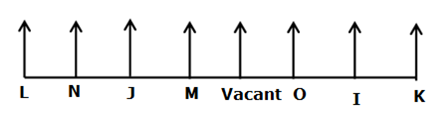1) J sits third from one of the ends.

2) Only two seats are there between J and O.

3) From the above condition, there are two possibilities.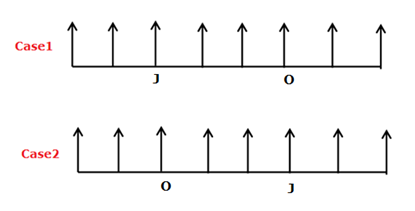4) M and O have only one neighbor. M sits adjacent to J.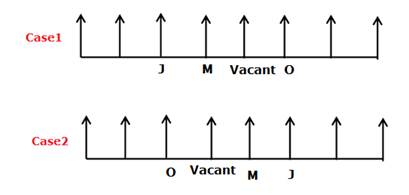5) The number of persons sitting between O and J are the same as between J and L.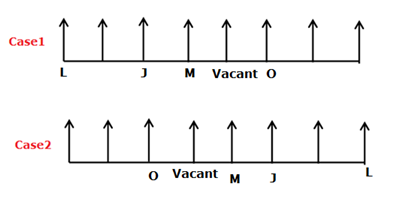6) The number of persons sitting left of N is the same as to the right of I.

7) K is not an immediate neighbor of N.

8) I sit right of O.

9) From the above condition, case2 gets eliminated. case1 shows the final arrangement.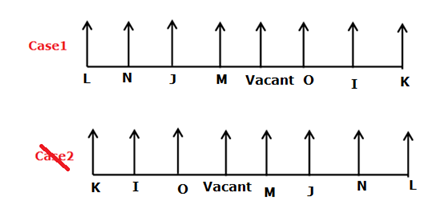Directions (6-8) :

B has a mobile which is costlier only than E.

_>_>_>_> B > E

C has a costlier mobile than F who has costlier mobile than D.

C > F > D

Only two persons are having mobile between C and A.

F has a costlier mobile than A.

C > F > D > A > B > E

Third costlier mobile rate is 45000.

C > F > D (45000) > A > B> E

LITERATURE

ERUTARETIL